Electricity Coulombs Law and Circuits Vocabulary Electric charge

• Slides: 75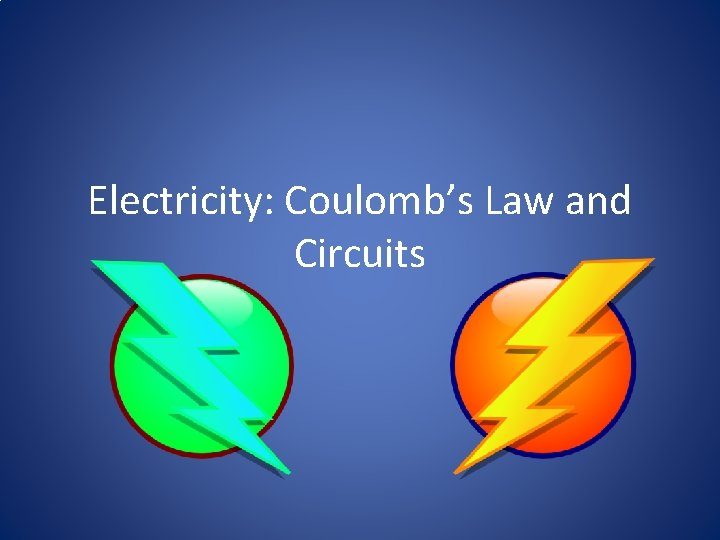Electricity: Coulomb’s Law and CircuitsVocabulary • Electric charge (q) – exists due to excess or deficient electrons on an object. Charge comes in two kinds: positive and negative. – The unit of charge is the coulomb. • Electric current (I) – is the flow of (positive) charge per second. – The unit of current are amperes. – One ampere means one coulomb of charge flowing per second.Vocabulary Contd. • Resistance (R) – tells how difficult it is for charge to flow through a circuit element. – measured in ohms (Ω) • Resistivity (ρ) – is a property of a material, which implies what the resistance would be of a meter-cube bit of that material. • Voltage (V) – is electrical potential energy per coulomb of charge.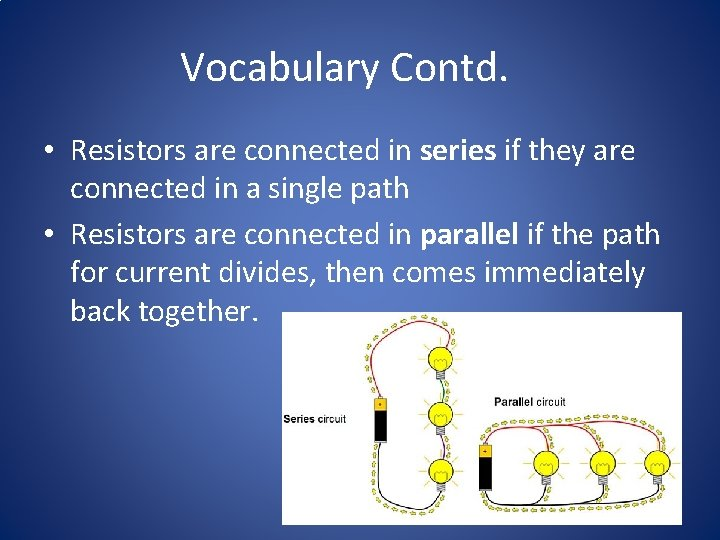Vocabulary Contd. • Resistors are connected in series if they are connected in a single path • Resistors are connected in parallel if the path for current divides, then comes immediately back together.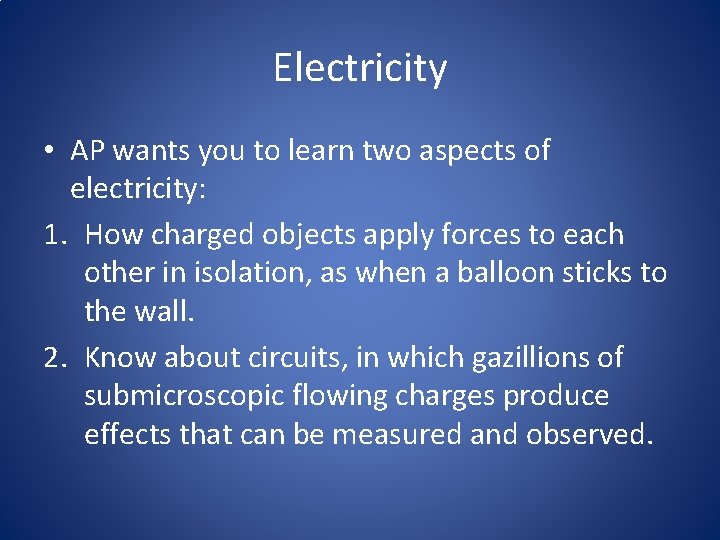Electricity • AP wants you to learn two aspects of electricity: 1. How charged objects apply forces to each other in isolation, as when a balloon sticks to the wall. 2. Know about circuits, in which gazillions of submicroscopic flowing charges produce effects that can be measured and observed.Electric Charge • All matter is made up of three types of particles: protons, neutrons, and electrons • Protons have an intrinsic property called “positive charge” • Neutrons don’t contain any charge • Electrons have a property called “negative charge”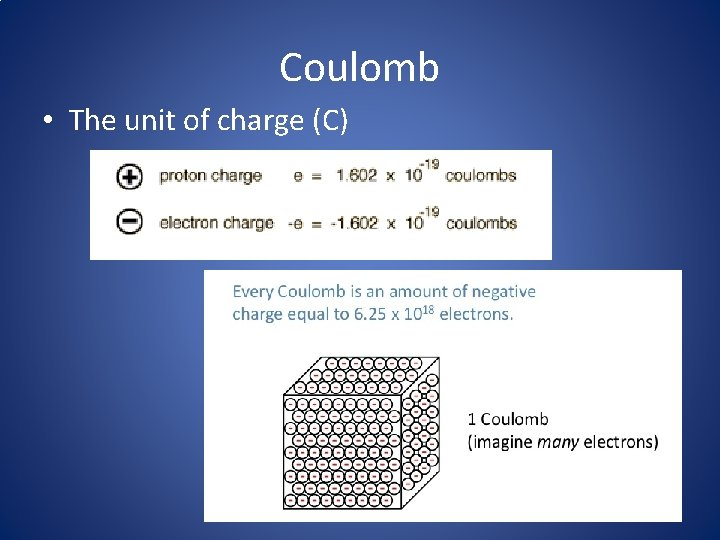Coulomb • The unit of charge (C)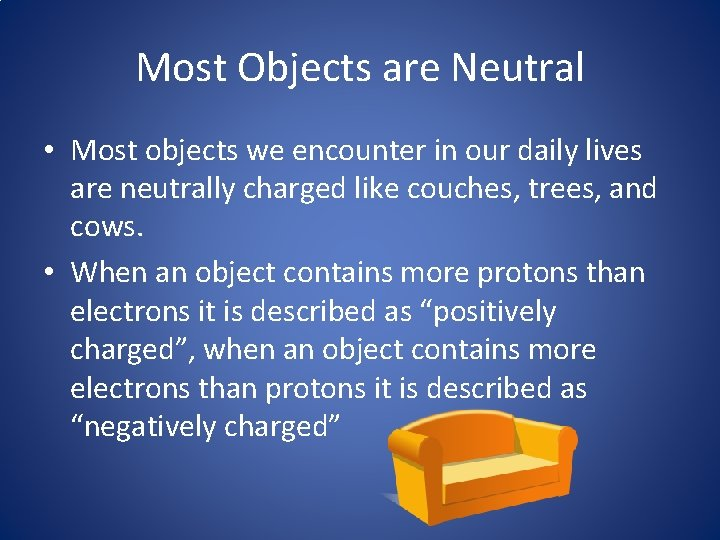Most Objects are Neutral • Most objects we encounter in our daily lives are neutrally charged like couches, trees, and cows. • When an object contains more protons than electrons it is described as “positively charged”, when an object contains more electrons than protons it is described as “negatively charged”Most Objects are Neutral • The reason big objects like couches, trees, and cows don’t behave like charged particles is because they contain so many bazillions of protons and electrons that an extra few here or there won’t really make much of a difference. • So even if they have a slight electric charge, it is too small, relatively speaking, to detect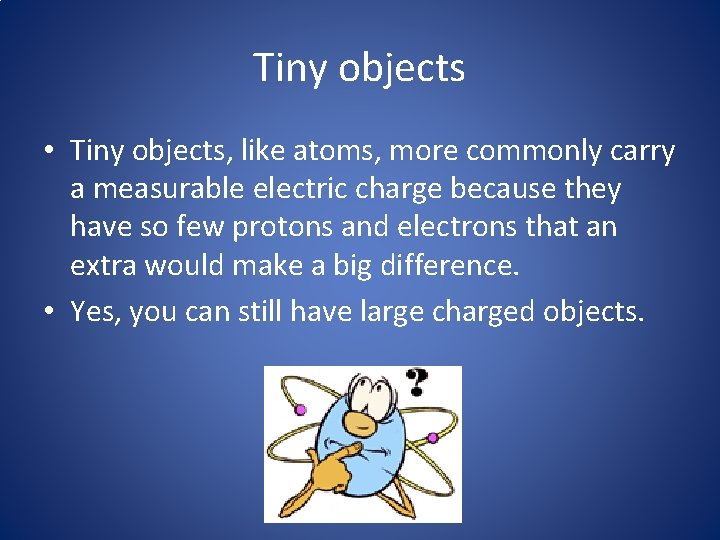Tiny objects • Tiny objects, like atoms, more commonly carry a measurable electric charge because they have so few protons and electrons that an extra would make a big difference. • Yes, you can still have large charged objects.Large charged objects • For instance, when you walk across a carpeted floor in the winter, you pick up lots of extra charges and become a charged object yourself… until you touch a doorknob • Then all the excess charge in your body travels through your finger and into the doorknob, causing you to feel a mild electric shock.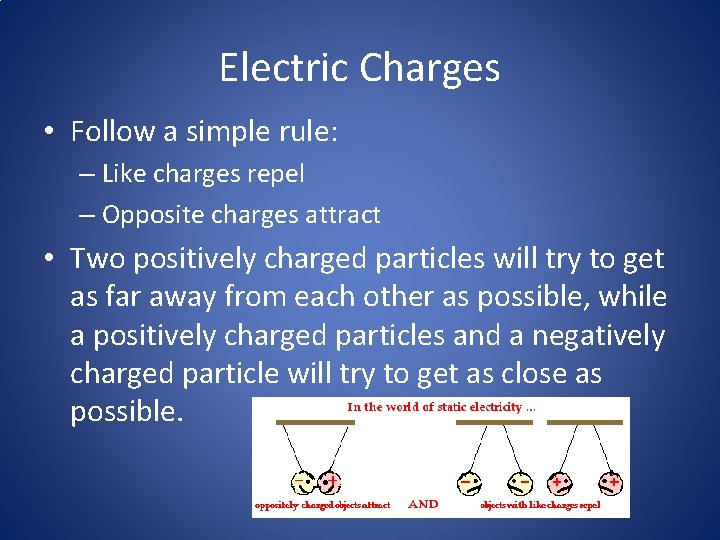Electric Charges • Follow a simple rule: – Like charges repel – Opposite charges attract • Two positively charged particles will try to get as far away from each other as possible, while a positively charged particles and a negatively charged particle will try to get as close as possible.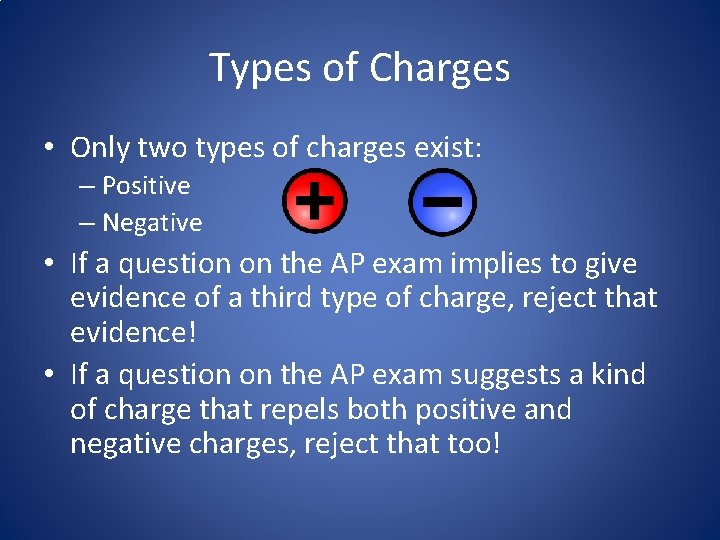Types of Charges • Only two types of charges exist: – Positive – Negative • If a question on the AP exam implies to give evidence of a third type of charge, reject that evidence! • If a question on the AP exam suggests a kind of charge that repels both positive and negative charges, reject that too!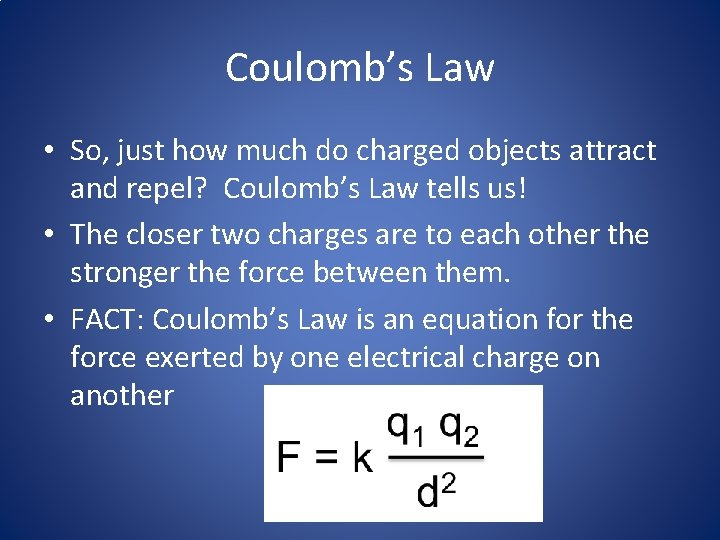Coulomb’s Law • So, just how much do charged objects attract and repel? Coulomb’s Law tells us! • The closer two charges are to each other the stronger the force between them. • FACT: Coulomb’s Law is an equation for the force exerted by one electrical charge on anotherCoulomb’s Law Contd. • The “qs” represent the amount of charge on each object • The “d” represents the distance between the two objects • Variable “k” is the Coulomb’s law constantAP Type Question • You will not be asked to calculate much with Coulomb’s law but the questions will be qualitative and semiquantitative. • Example 1, if the amount of charge A is doubled, what happens to the force of charge A on charge B? • Example 2, if you double the distance between two charges, what happens to the force of one on the other?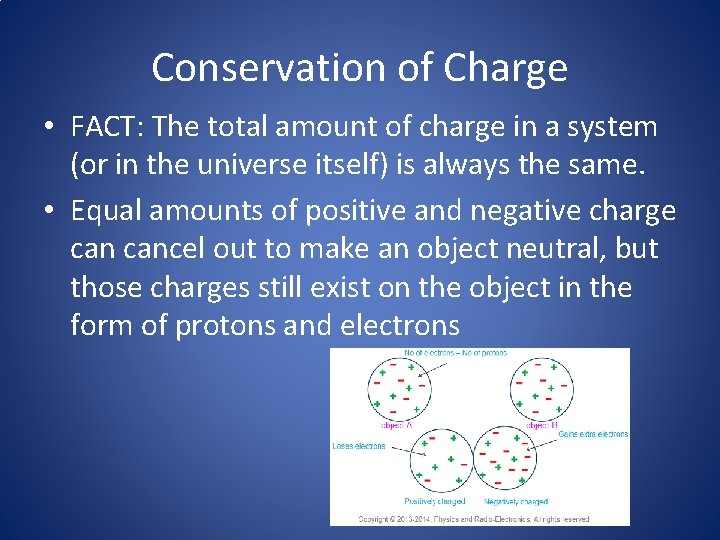Conservation of Charge • FACT: The total amount of charge in a system (or in the universe itself) is always the same. • Equal amounts of positive and negative charge cancel out to make an object neutral, but those charges still exist on the object in the form of protons and electrons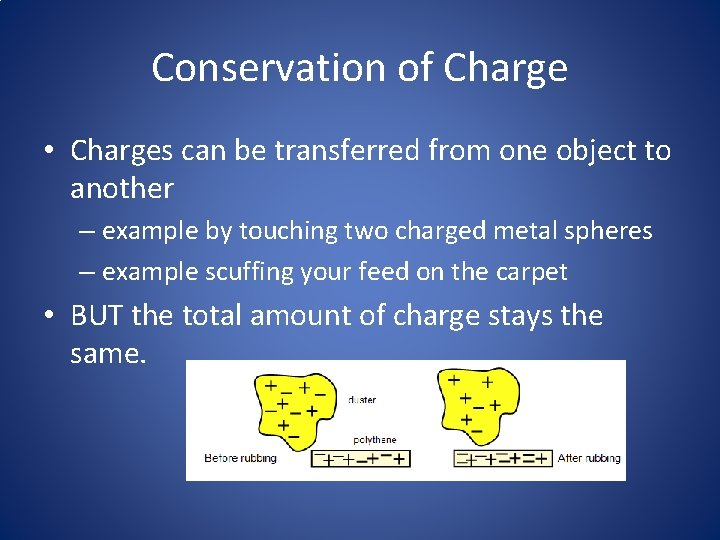Conservation of Charge • Charges can be transferred from one object to another – example by touching two charged metal spheres – example scuffing your feed on the carpet • BUT the total amount of charge stays the same.Circuits • A circuit is a wire path that allows charge to flow. • A current is defined as the flow of positive charge • Under what conditions would this charge flow through a wire?Circuits • It occurs when a coulomb of charge has a potential energy that’s higher at one position in the wire than the other. • We call this difference in potential energy per coulomb a “voltage” and a battery’s job is to provide this voltage that allows current to flow. • Current flows out of a battery from the positive side of the battery to the negative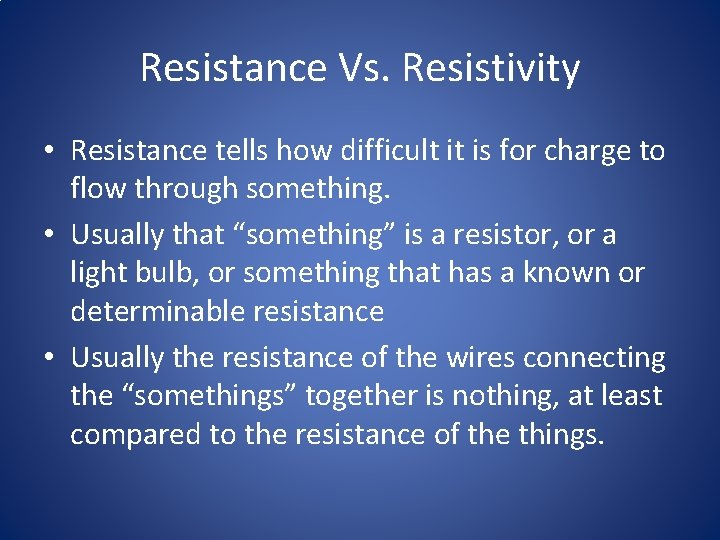Resistance Vs. Resistivity • Resistance tells how difficult it is for charge to flow through something. • Usually that “something” is a resistor, or a light bulb, or something that has a known or determinable resistance • Usually the resistance of the wires connecting the “somethings” together is nothing, at least compared to the resistance of the things.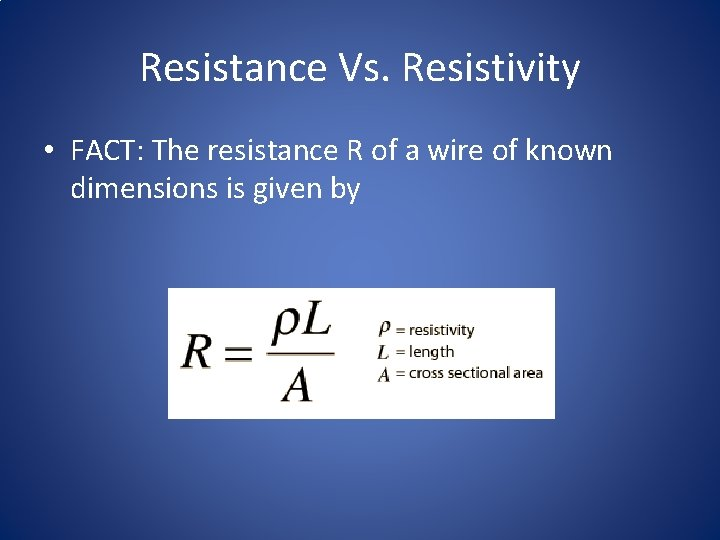Resistance Vs. Resistivity • FACT: The resistance R of a wire of known dimensions is given byResistance Vs. Resistivity • The longer the wire is, the more its resistance • The “wider” the wire is the less its resistance – That is the bigger its cross-sectional area • Two wires with the same shapes can have different resistances if they are made of different materials • Assuming the same shape, the wire with more resistance has a greater resistivity, represented by the variable ρ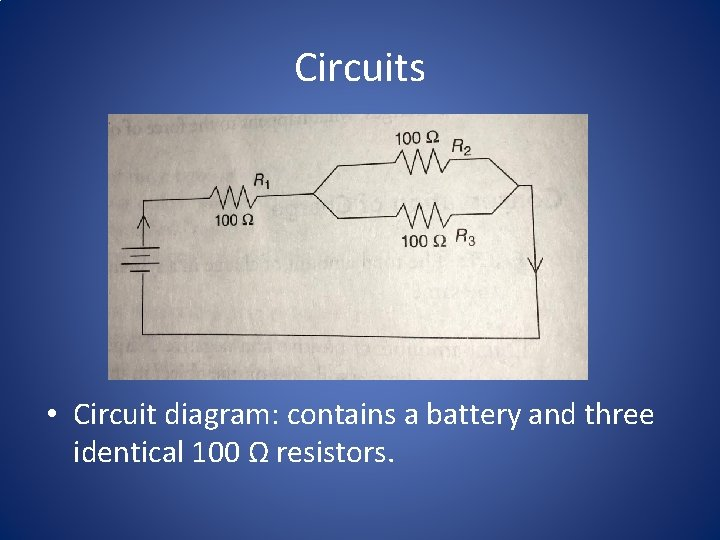Circuits • Circuit diagram: contains a battery and three identical 100 Ω resistors.What will AP ask? • Questions about circuits will occasionally ask for calculation: – Find the voltage across each resistor. – Find the current through each resistor. • More often you’ll be asked qualitative questions – Which bulb takes the greatest current? – Rank resistors from largest to smallest voltage across.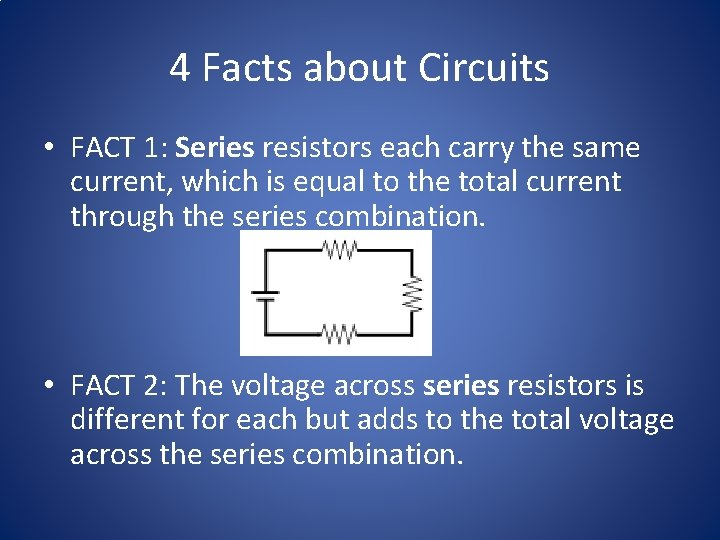4 Facts about Circuits • FACT 1: Series resistors each carry the same current, which is equal to the total current through the series combination. • FACT 2: The voltage across series resistors is different for each but adds to the total voltage across the series combination.4 Facts about Circuits • FACT 3: The voltage across parallel resistors is the same for each and equal to the total voltage across the parallel combination • FACT 4: Parallel resistors each carry different currents, which add to the total current through the parallel combination.AP Exam Tip • If you are confused by a qualitative circuit question, try answering with a calculation: – “Well, with a 150 -V battery here’s a calculation showing that I get 1 A of current in the circuit, but with a 75 -V battery I only get 0. 5 A. Thus, cutting the battery’s voltage in half also cuts the current in half. ”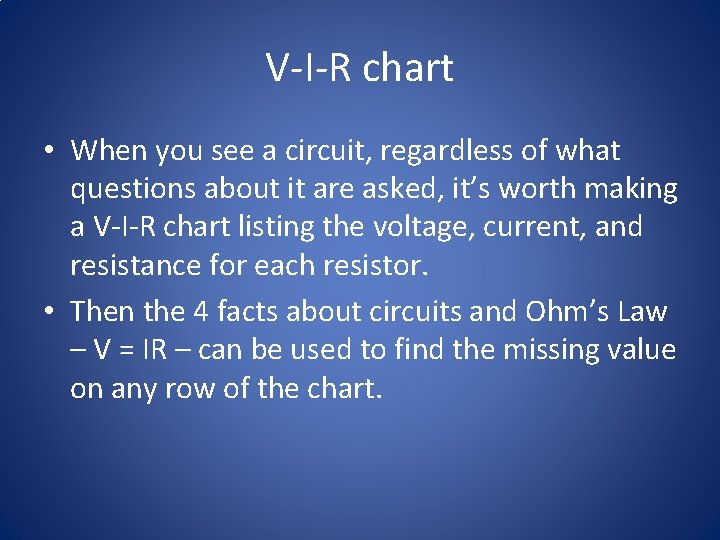V-I-R chart • When you see a circuit, regardless of what questions about it are asked, it’s worth making a V-I-R chart listing the voltage, current, and resistance for each resistor. • Then the 4 facts about circuits and Ohm’s Law – V = IR – can be used to find the missing value on any row of the chart.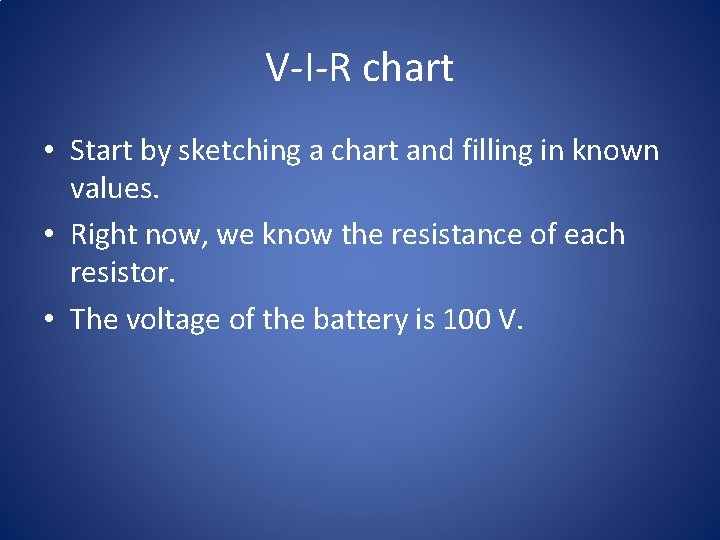V-I-R chart • Start by sketching a chart and filling in known values. • Right now, we know the resistance of each resistor. • The voltage of the battery is 100 V.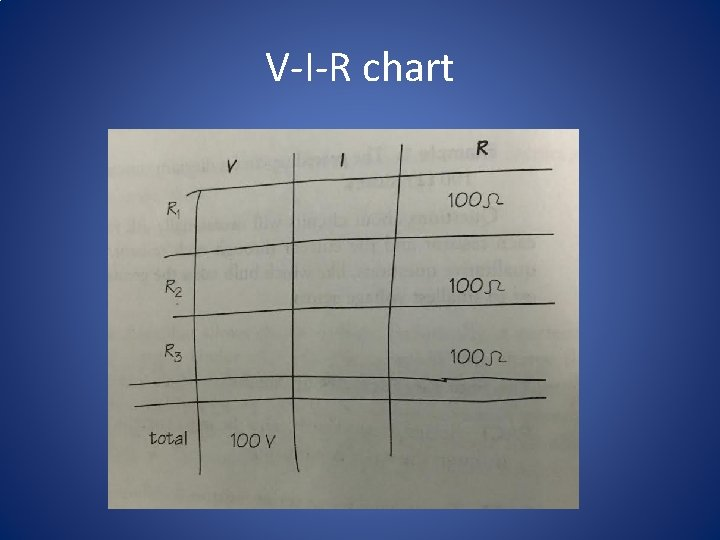V-I-R chart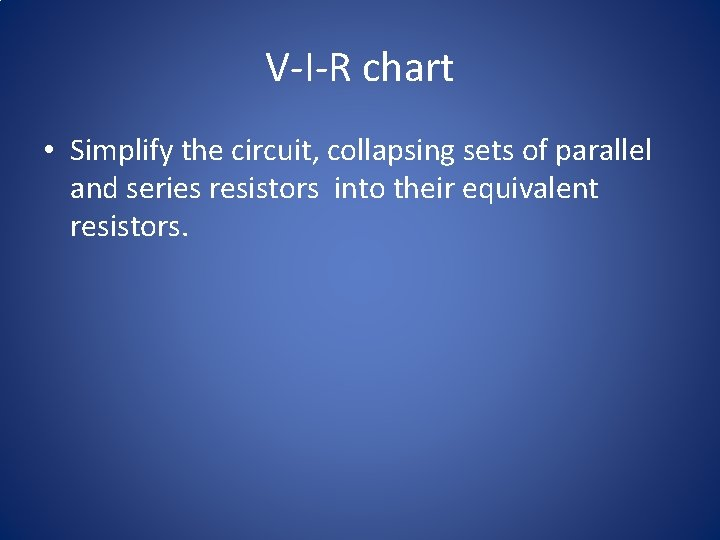V-I-R chart • Simplify the circuit, collapsing sets of parallel and series resistors into their equivalent resistors.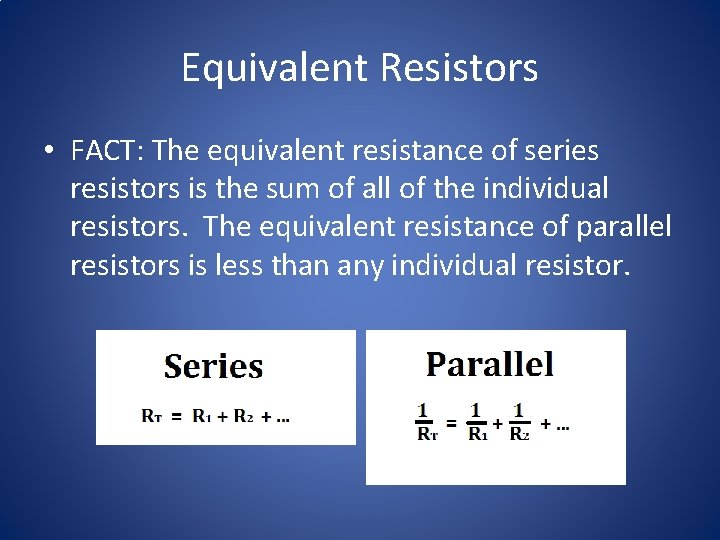Equivalent Resistors • FACT: The equivalent resistance of series resistors is the sum of all of the individual resistors. The equivalent resistance of parallel resistors is less than any individual resistor.Equivalent Resistors • You can use this equation to find the equivalent resistance of resistors that are in parallel: • Here, then, the parallel combination of resistors has equivalent resistance of 50 Ω.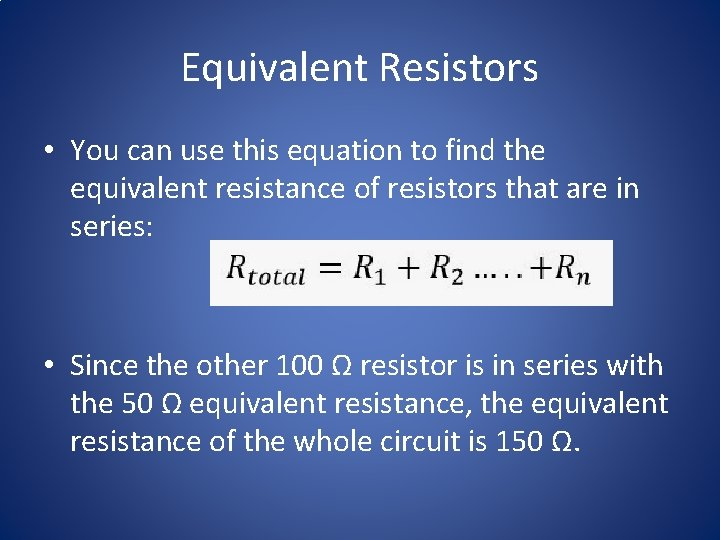Equivalent Resistors • You can use this equation to find the equivalent resistance of resistors that are in series: • Since the other 100 Ω resistor is in series with the 50 Ω equivalent resistance, the equivalent resistance of the whole circuit is 150 Ω.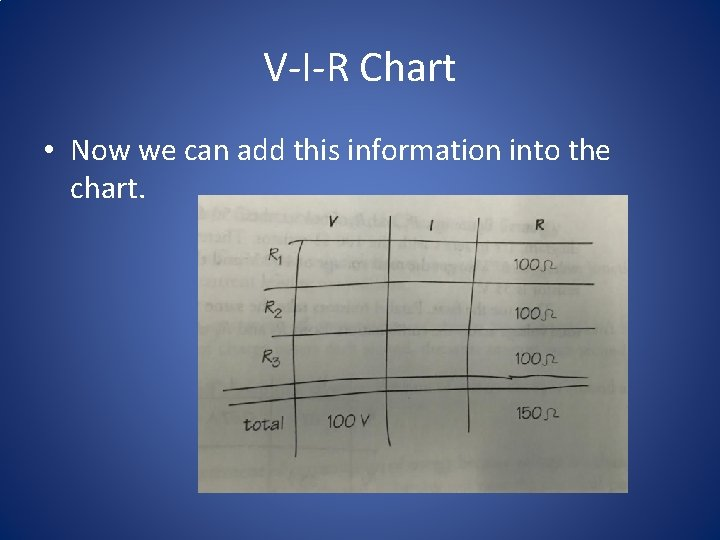V-I-R Chart • Now we can add this information into the chart.MISTAKE! • The V-I-R Chart is not a magic square, it’s a tool for organizing your calculations for a complicated circuit. • You can NOT just add values up and down the columns.Ohm’s Law • Now that we have made progress and two of the three entries in the “total” row are complete. • Therefore, we can use Ohm’s Law to calculate the total current in this circuit.Ohm’s Law • FACT: Ohm’s law says that voltage across a circuit element equals that element’s current times its resistance.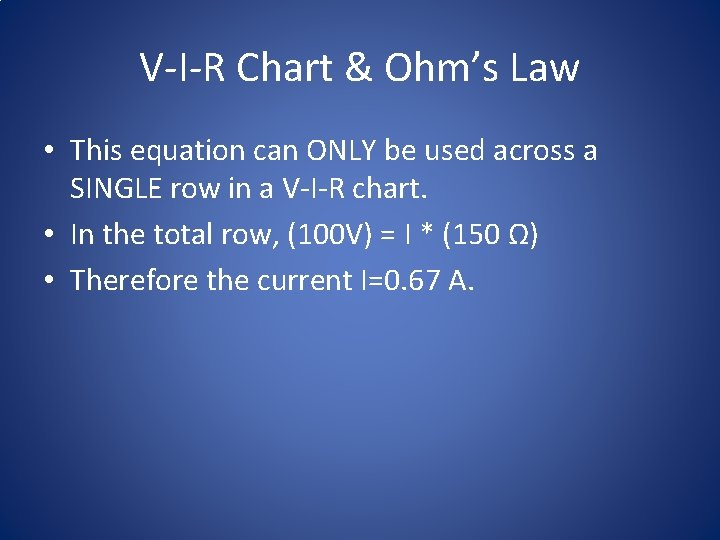V-I-R Chart & Ohm’s Law • This equation can ONLY be used across a SINGLE row in a V-I-R chart. • In the total row, (100 V) = I * (150 Ω) • Therefore the current I=0. 67 A.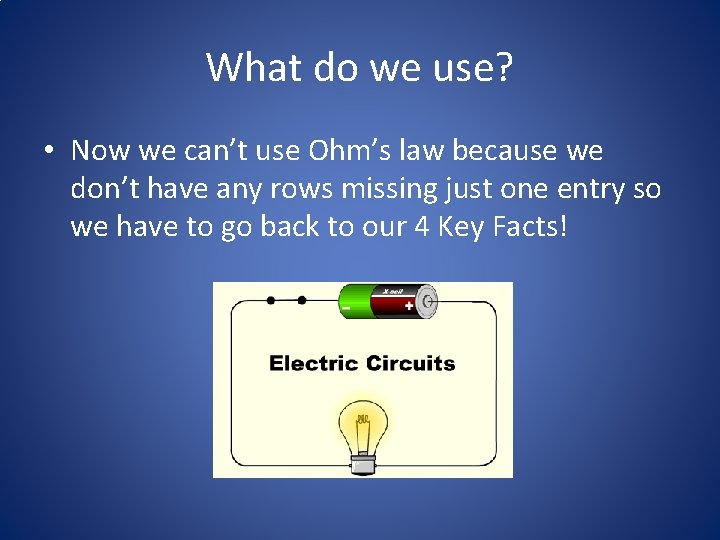What do we use? • Now we can’t use Ohm’s law because we don’t have any rows missing just one entry so we have to go back to our 4 Key Facts!Using the Facts • Resistor R 1 is in series with the battery; since current through series resistors is equal to the total current, R 1 must take the entire current flowing from the battery, all 0. 67 A. • AHA! Now you can put 0. 67 A in the chart for the current through R 1, and we can use Ohm’s Law to calculate the voltage across R 1; that’s 67 V.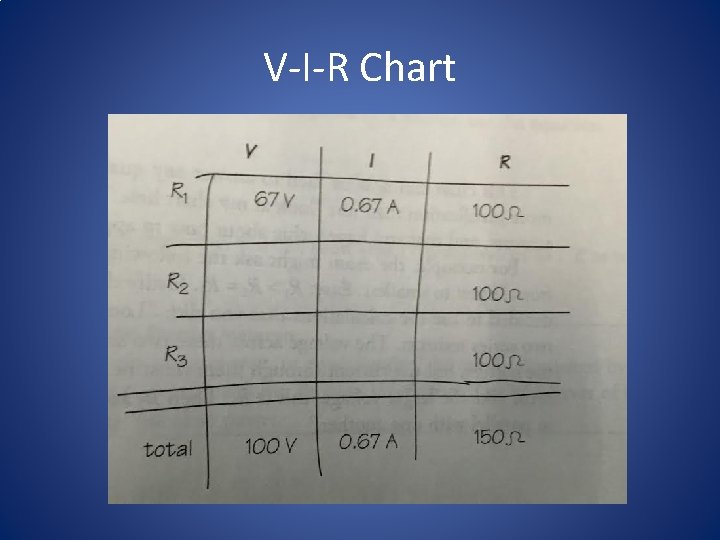V-I-R Chart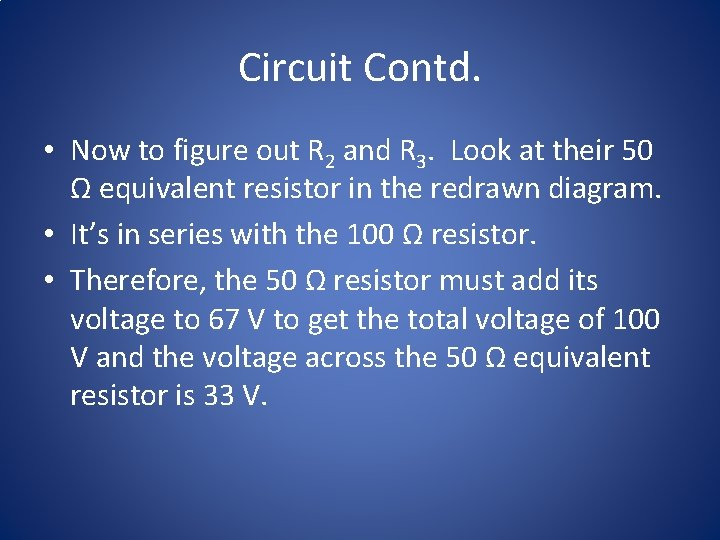Circuit Contd. • Now to figure out R 2 and R 3. Look at their 50 Ω equivalent resistor in the redrawn diagram. • It’s in series with the 100 Ω resistor. • Therefore, the 50 Ω resistor must add its voltage to 67 V to get the total voltage of 100 V and the voltage across the 50 Ω equivalent resistor is 33 V.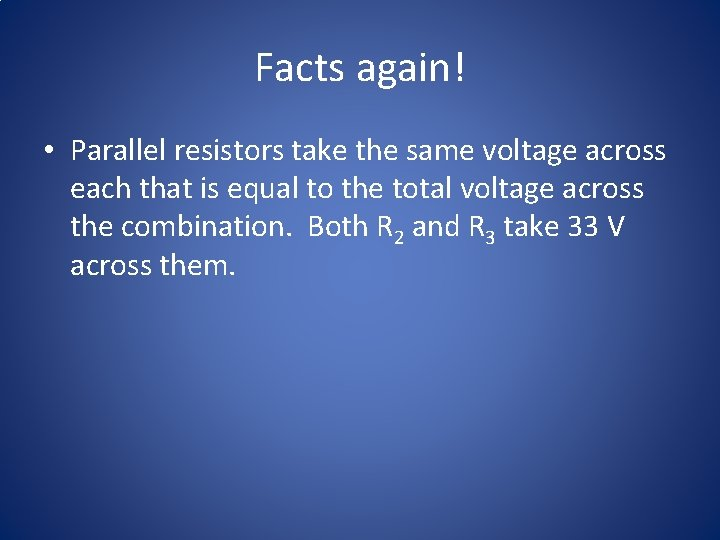Facts again! • Parallel resistors take the same voltage across each that is equal to the total voltage across the combination. Both R 2 and R 3 take 33 V across them.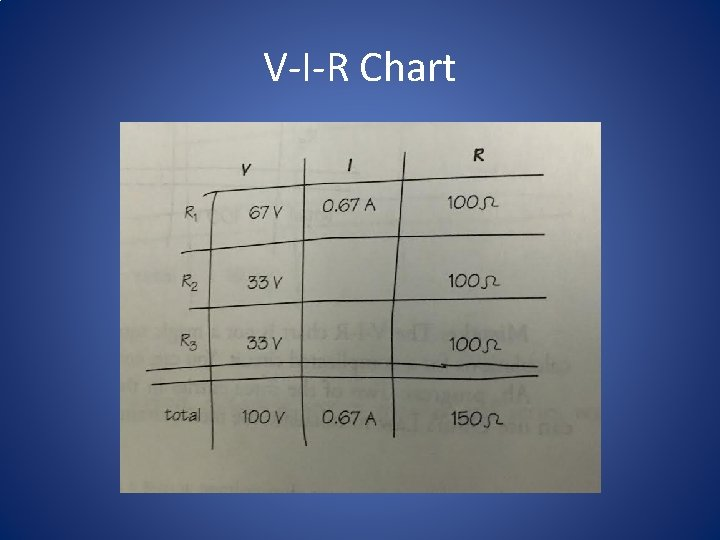V-I-R Chart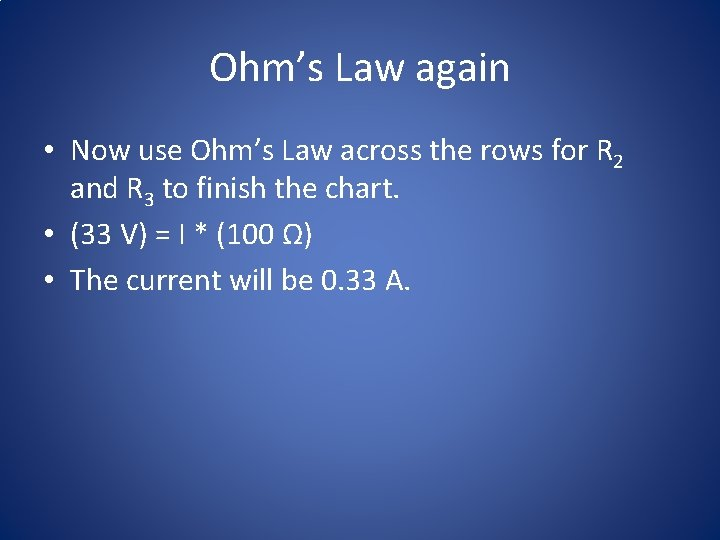Ohm’s Law again • Now use Ohm’s Law across the rows for R 2 and R 3 to finish the chart. • (33 V) = I * (100 Ω) • The current will be 0. 33 A.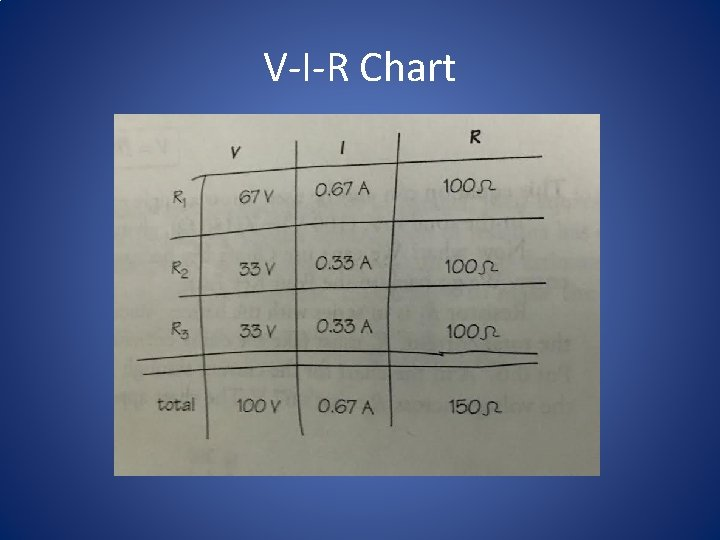V-I-R ChartV-I-R Chart • Now the chart can be used to answer any qualitative question. • Yes, you need to give more justification then “hey, look at my chart!” • The chart will ensure you get the right answers, and that you have a clue about how to approach the qualitative questions.AP Type Question • Example, the exam might ask the following: – Rank the voltage across each resistor from largest to smallest. – Justify your answer.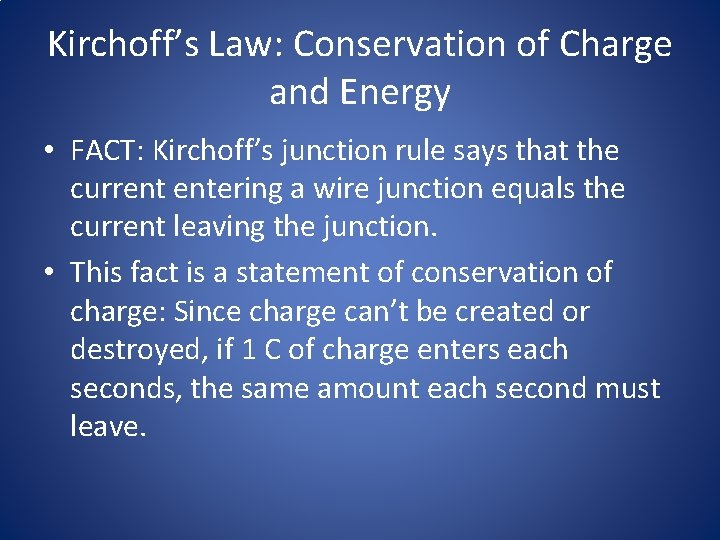Kirchoff’s Law: Conservation of Charge and Energy • FACT: Kirchoff’s junction rule says that the current entering a wire junction equals the current leaving the junction. • This fact is a statement of conservation of charge: Since charge can’t be created or destroyed, if 1 C of charge enters each seconds, the same amount each second must leave.Kirchoff’s Law: Conservation of Charge and Energy • FACT: Kirchoff’s loop rule says that the sum of voltage changes around a circuit loop is zero. • This fact is a statement of conservation of energy because voltage is a change in the electrical potential energy of 1 C of charge. A battery can raise the electrical potential energy of some charge that flows; a resistor will lower the potential energy of that charge. But the sum of all these energy changes must be zero.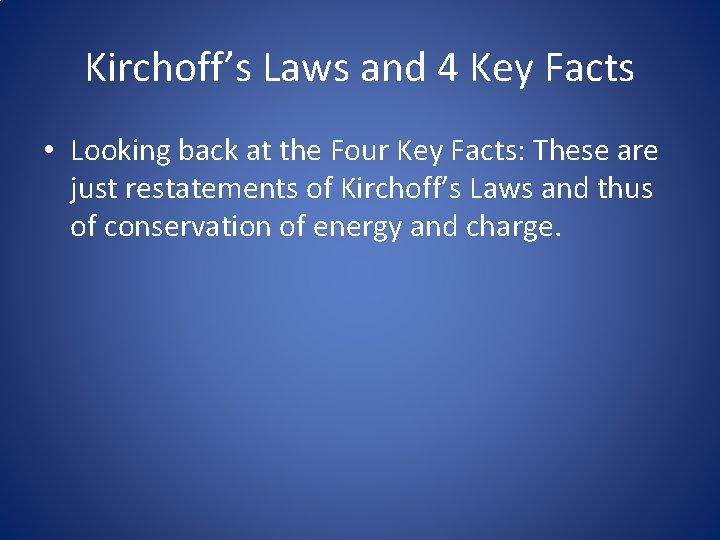Kirchoff’s Laws and 4 Key Facts • Looking back at the Four Key Facts: These are just restatements of Kirchoff’s Laws and thus of conservation of energy and charge.Junction Rule • The junction rule applies to the facts about current. • Series resistors take the same current through each, because there’s no junction. • The current through parallel resistors adds to the total because of the junction before and after the parallel combination.Junction Rule • Conservation of chargeLoop Rule • The loop rule applies to the facts about voltage. • The voltage across series resistors adds to the total voltage because the resistors can only drop the potential energy of 1 C of charge as much as the battery raised the charge’s potential energy.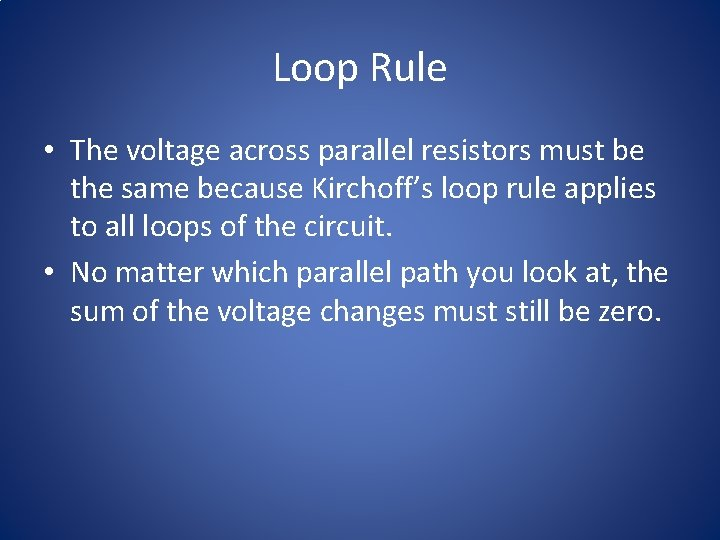Loop Rule • The voltage across parallel resistors must be the same because Kirchoff’s loop rule applies to all loops of the circuit. • No matter which parallel path you look at, the sum of the voltage changes must still be zero.Loop Rule • Conservation of energyPower in a Circuit • Power is defined as energy per second. • Resistors generally convert electrical energy to other forms of energy • The amount of power says how quickly that conversion occurs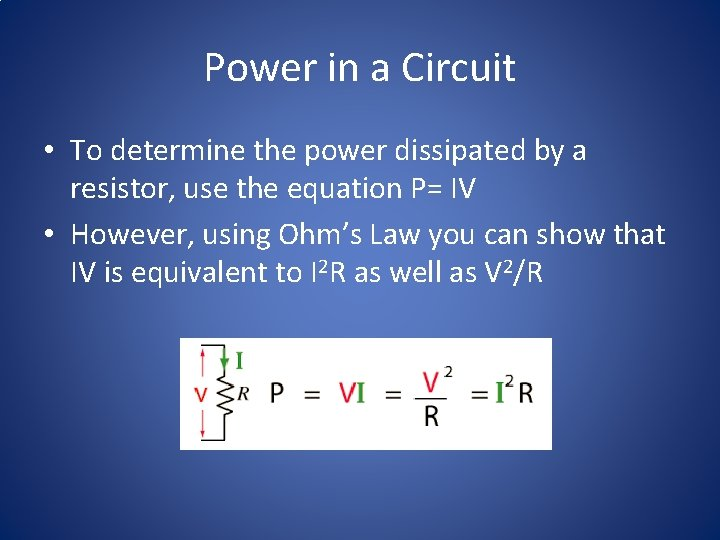Power in a Circuit • To determine the power dissipated by a resistor, use the equation P= IV • However, using Ohm’s Law you can show that IV is equivalent to I 2 R as well as V 2/R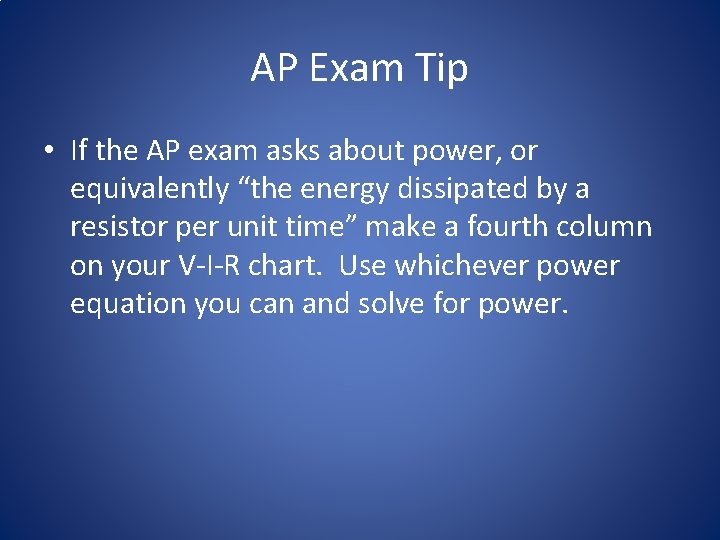AP Exam Tip • If the AP exam asks about power, or equivalently “the energy dissipated by a resistor per unit time” make a fourth column on your V-I-R chart. Use whichever power equation you can and solve for power.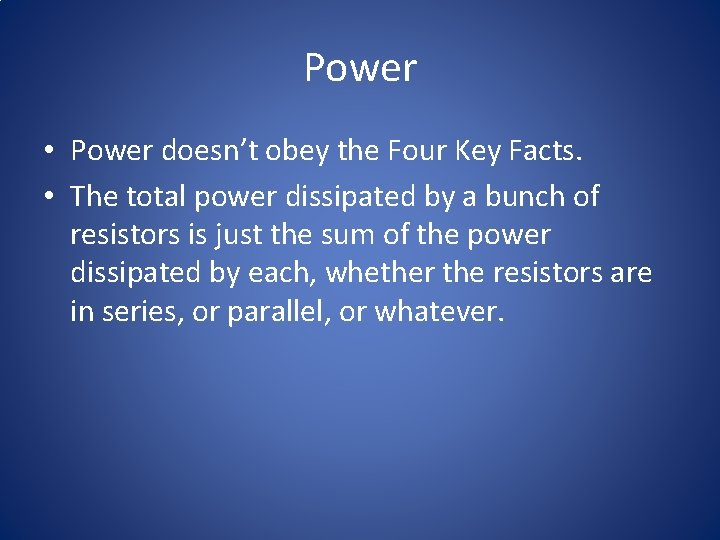Power • Power doesn’t obey the Four Key Facts. • The total power dissipated by a bunch of resistors is just the sum of the power dissipated by each, whether the resistors are in series, or parallel, or whatever.Circuits: Experimental Point of View • When a real circuit is set up in the laboratory, it usually consists of more than just resistors – light bulbs and motors are common devices to hook to a battery. • For the purposes of computation, though, we can consider pretty much any electronic device to act like a resistor.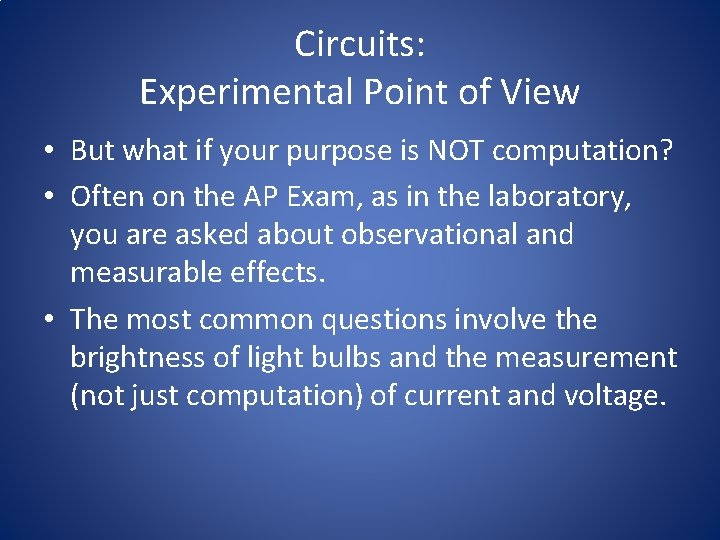Circuits: Experimental Point of View • But what if your purpose is NOT computation? • Often on the AP Exam, as in the laboratory, you are asked about observational and measurable effects. • The most common questions involve the brightness of light bulbs and the measurement (not just computation) of current and voltage.Brightness of a Bulb • The brightness of a bulb depends solely on the power dissipated by the bulb. • Remember the power equations • The bulb’s power can change depending on the current and voltage it’s hooked up to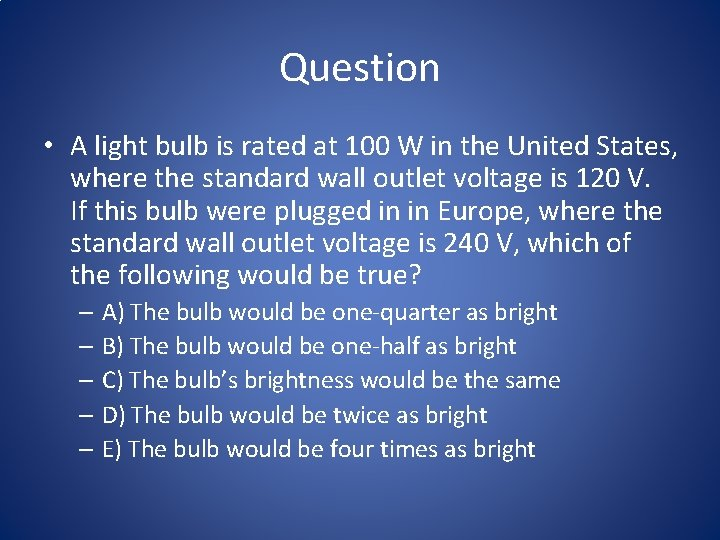Question • A light bulb is rated at 100 W in the United States, where the standard wall outlet voltage is 120 V. If this bulb were plugged in in Europe, where the standard wall outlet voltage is 240 V, which of the following would be true? – A) The bulb would be one-quarter as bright – B) The bulb would be one-half as bright – C) The bulb’s brightness would be the same – D) The bulb would be twice as bright – E) The bulb would be four times as bright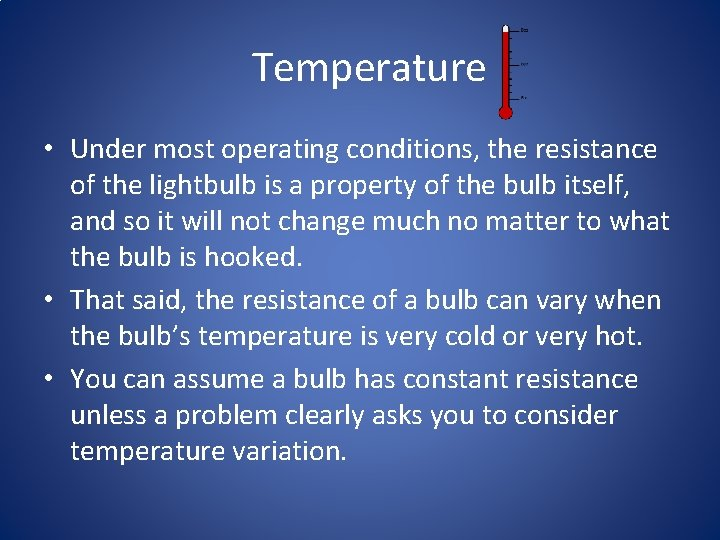Temperature • Under most operating conditions, the resistance of the lightbulb is a property of the bulb itself, and so it will not change much no matter to what the bulb is hooked. • That said, the resistance of a bulb can vary when the bulb’s temperature is very cold or very hot. • You can assume a bulb has constant resistance unless a problem clearly asks you to consider temperature variation.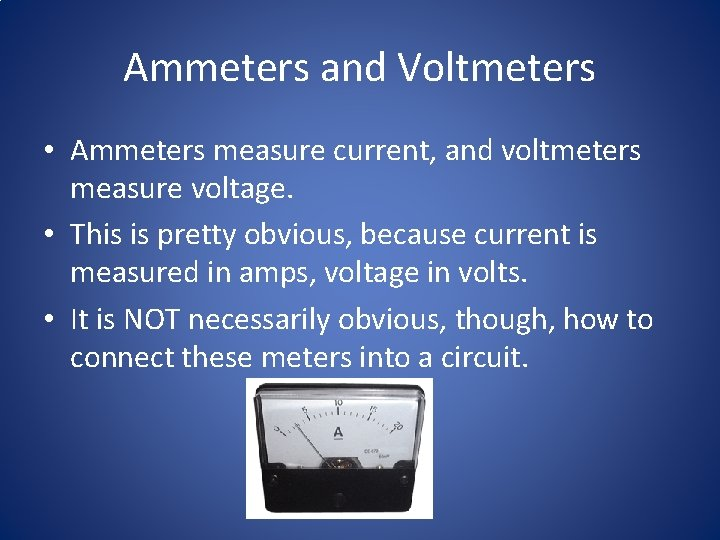Ammeters and Voltmeters • Ammeters measure current, and voltmeters measure voltage. • This is pretty obvious, because current is measured in amps, voltage in volts. • It is NOT necessarily obvious, though, how to connect these meters into a circuit.Ammeters and Voltmeters • Remind yourself of the properties of series and parallel resistors • Voltage is the same for any resistors in parallel with each other • So if you’re going to measure the voltage across a resistor, you must put the voltmeter in parallel with the resistor.Ammeters and VoltmetersAmmeters and Voltmeters • In the picture, the meter labeled V 2 measures the voltage across the 100 Ω resistor, while the meter labeled V 1 measures the potential difference between points A and B (which is also the voltage across R 1).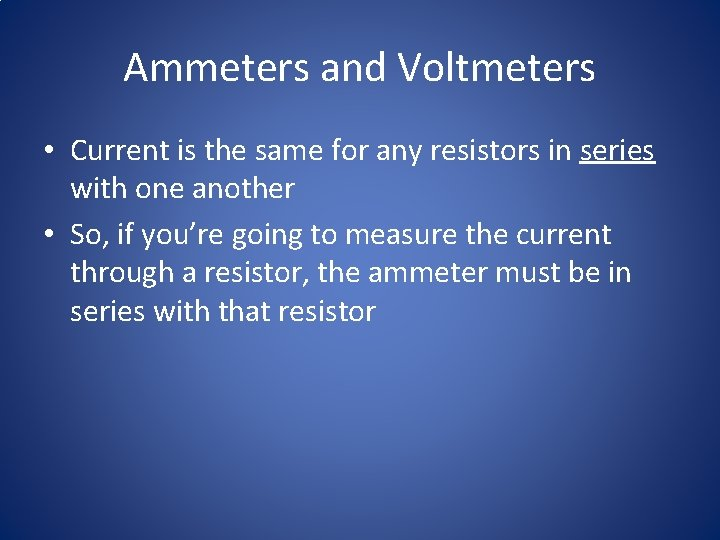Ammeters and Voltmeters • Current is the same for any resistors in series with one another • So, if you’re going to measure the current through a resistor, the ammeter must be in series with that resistor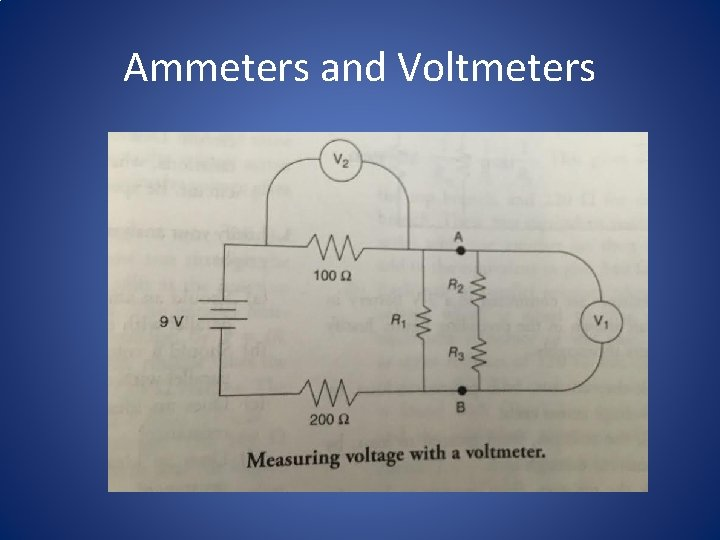Ammeters and VoltmetersAmmeters and Voltmeters • Ammeter A 1 measures the current through resistor R 1, while ammeter A 2 measures the current through resistor R 2. • Is there a way to figure out the current in the other three resistors based on the readings in these two ammeters?